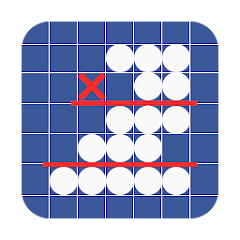# 筆算電卓

5K+Everyone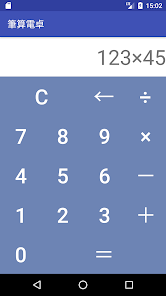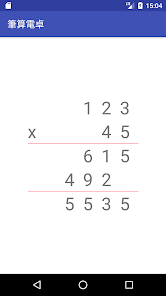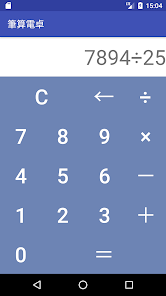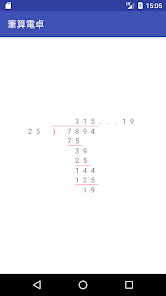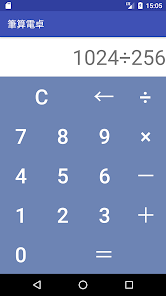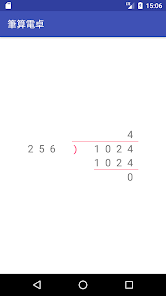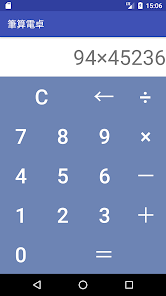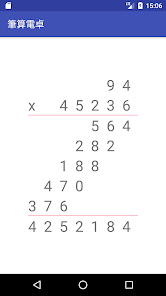Is a calculator that calculation results are displayed along with the "longhand".

Only displays the answer that it is a normal calculator.
I tried to calculate the multiplication and division in longhand,
If you disagree with the answer of the calculator,
I think you worried How is wrong to in the course of longhand?
Please use this longhand calculator when such.
Since longhand and the answer is displayed, you know immediately where you were wrong.

Division is also displayed "too much".
Please use the "longhand calculator" even if you want to find the "remainder".

■ What you can do with "longhand calculator"
subtraction
multiplication
Division (to display the answer in the remainder)

To answer alignment of elementary school math longhand of a problem.
To answer alignment of the cram school and Kumon.
Out to the division of the remainder.
Also of interest when the teachers to create a math test problem.
Updated on
Oct 5, 2018

## Data safety